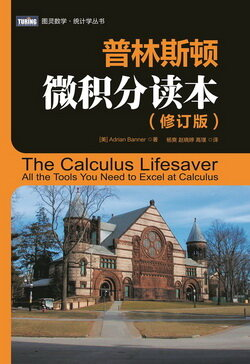# 普林斯顿微积分读本（修订版）(15)：三角学回顾 2&2.1(三角学回顾)

• 用弧度度量的角与三角函数的基本知识 ;
• 实轴上的三角函数 (不只是介于 0° 和 90° 的角);
• 三角函数的图像 ;
• 三角恒等式.

(基本知识)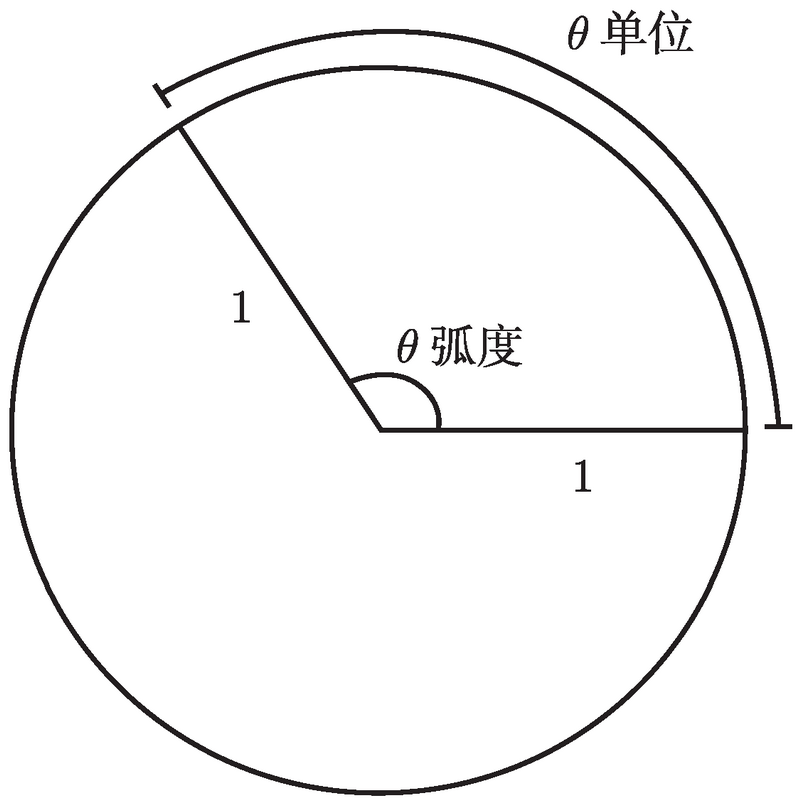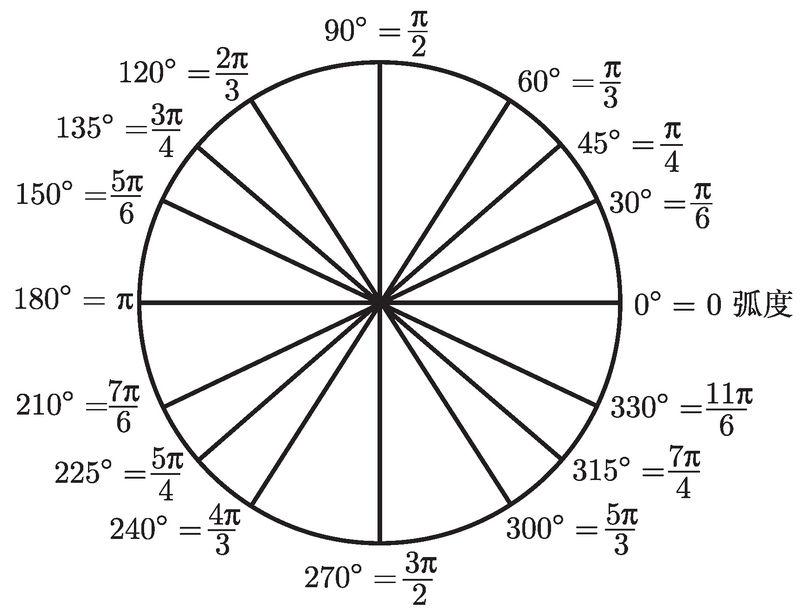$\frac{5\pi}{12}=\frac{\pi}{180}\times$ 用度计量的角,

sin (θ) =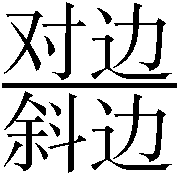,　cos (θ) =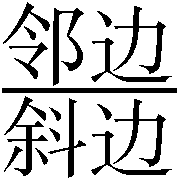,　tan (θ) =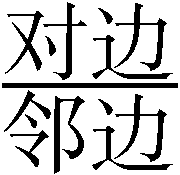.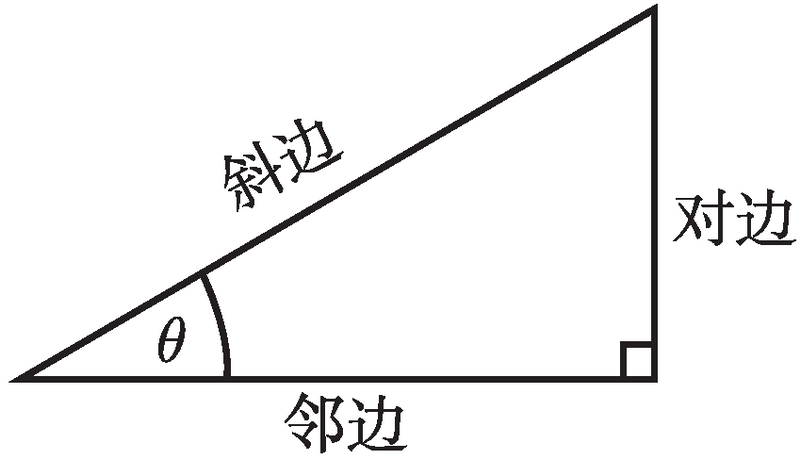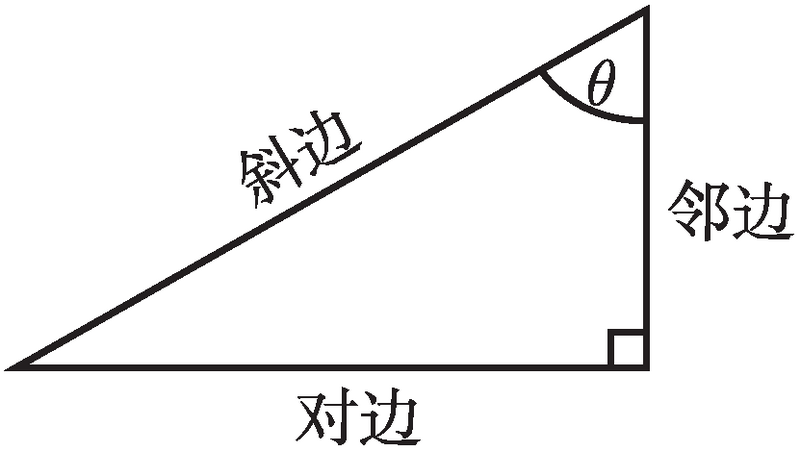$\csc(x)=\frac{1}{\sin(x)},\quad\sec(x)=\frac{1}{\cos(x)},\quad\cot(x)=\frac{1}{\tan(x)}.$

0 $\frac{\pi}{6}$ $\frac{\pi}{4}$ $\frac{\pi}{3}$ $\frac{\pi}{2}$
sin 0 $\frac{1}{2}$ $\frac{1}{\sqrt{2}}$ $\frac{\sqrt{3}}{2}$ 1
cos 1 $\frac{\sqrt{3}}{2}$ $\frac{1}{\sqrt{2}}$ $\frac{1}{2}$ 0
tan 0 $\frac{1}{\sqrt{3}}$ 1 $\sqrt{3}$

(1) sin (π/3) 是什么？(使用该表, 答案是 $\sqrt{3}/2$. )

(2) 介于 0 到 π/2, 其正弦值为 $\sqrt{3}/2$ 的角是什么？(显然, 答案是 π/3. )